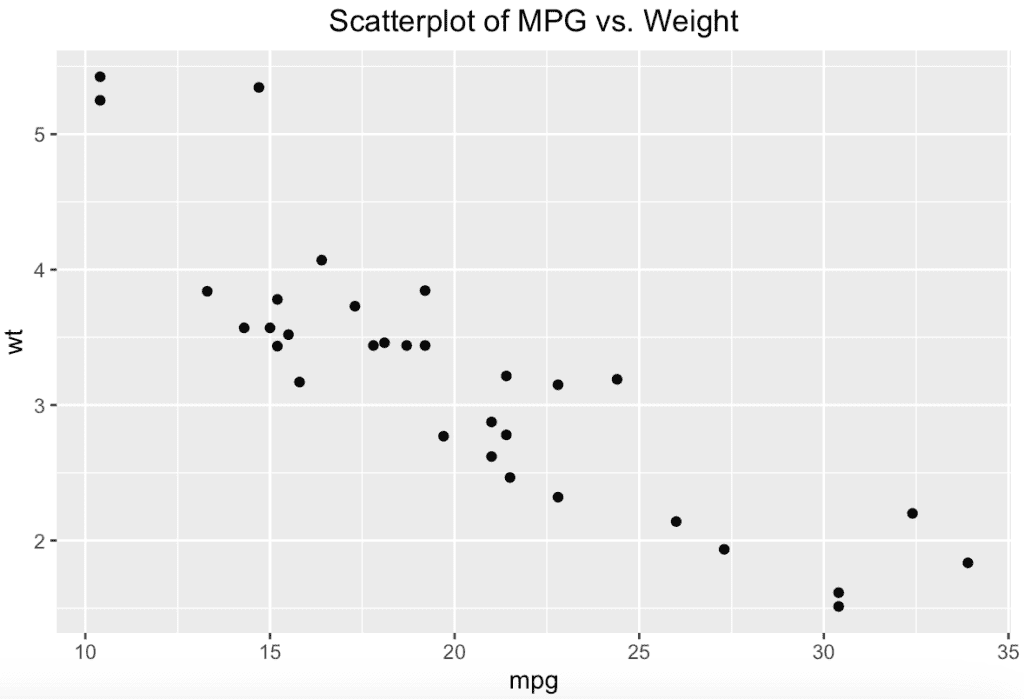# How to Center the Plot Title in ggplot2

Here are the steps to center the plot title in ggplot2:

1. Create a “ggplot” object.
2. Use the “plot title” to explain the main findings.
3. Use the “theme()” function and specify the argument “hjust = 0.5” in the “element_text()” function.

## Example

``````# Load required packages
library(ggplot2)

# Create a simple ggplot2 plot
plt <- ggplot(mtcars, aes(x = mpg, y = wt)) +
geom_point() +
labs(title = "Scatterplot of MPG vs. Weight") +
theme(plot.title = element_text(hjust = 0.5))

# Print the plot
print(plt)``````

OutputIn this example, we created a scatterplot of the mtcars dataset, displaying miles per gallon (MPG) against weight (WT).

We set the plot title using the labs() function and then adjust the title’s horizontal justification (hjust) inside the theme() function. Setting hjust = 0.5 centers the title.

Here’s a breakdown of the code:

1. Loaded the ggplot2 package with the library(ggplot2).
2. Created a scatterplot with ggplot() and geom_point() functions using the mtcars dataset.
3. Added a plot title with the labs() function.
4. Adjusted the plot title’s horizontal justification using the theme() function and setting the plot.title = element_text(hjust = 0.5).
5. Print the plot with the print() function.

That’s it.